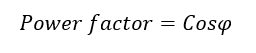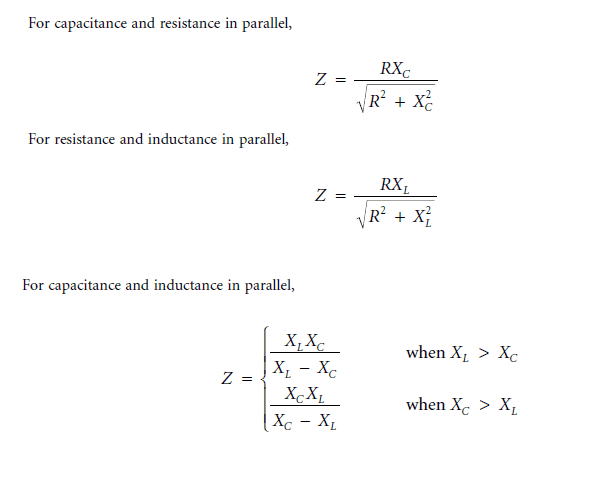# What is Impedance Reactance Impedance Triangle

## Impedance:

The total opposition to an alternating current circuit is called impedance. The opposition is created by resistor, inductor, and capacitor.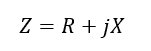Impedance is symbolized by “Z”. and the SI units of impedance is ohm (Ω). Also the name impedance conveys the notion that Z “impedes” phase current I, just like how resistance R resists the instantaneous current i

In AC circuits impedance Z is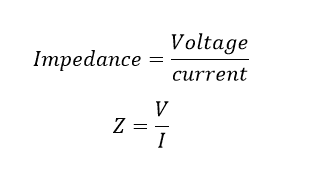## Reactance (X):

Reactance in a circuit is the opposition to an alternating current caused by inductance and capacitance, equal to the difference between capacitive and inductive reactance. Expressed in 0HMS.

### Inductive reactance (XL)

inductive reactance is that element of reactance in a circuit caused by self-inductance. Inductive reactance is united in ohms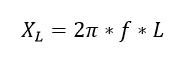Here L is the inductance of the coil In Henry

### Capacitance reactance (XC)

Capacitive reactance is that element of reactance in a circuit caused by capacitance. Capacitive reactance is united as ohms.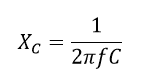Here C is the Capacitance of the coil In ohms.

## Impedance Triangle: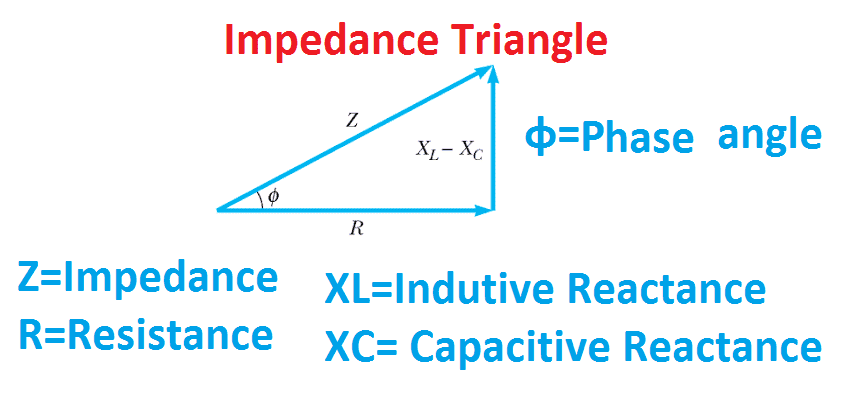Generally, the impedance can be calculated from the impedance triangle. From the triangle, The impedance Z is

Here the inductive reactance is greater than capacitive reactance. XL > XC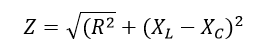Here the than capacitive reactance is greater than inductive reactance. XC > XL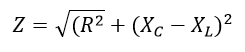For resistance the impedance is pure resistive and the reactance is zero whereas for capacitor and inductor the impedances are purely reactive and the resistance is zero.

For pure resistive circuit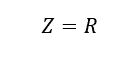For Pure inductive circuit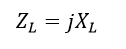For Pure Capacitive circuit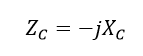Impedance of ZL and ZC are frequency independent. At low frequency when ω=0, then ZL=0 and Zc= ∞ which corresponds to dc steady state condition. This is the reason the inductor act as a short circuit and capacitor act as open circuit. For high frequency ω=∞ then Zc =0 and ZL= ∞. Then the capacitor act as a short circuit and inductor act as open circuit.

## Phase angle:

The phase angle is the phase difference between the voltage applied to the impedance and the current driven through it. Phase angle gives the time relationship between the current in the circuit and the voltage. It is denoted by ϕ. The phase angle is mathematically expressed by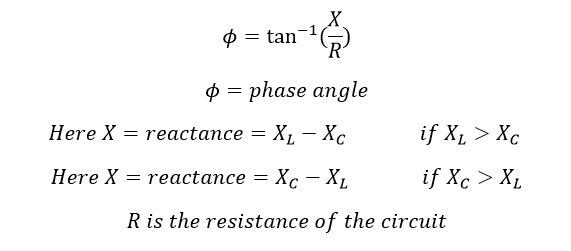Cosine of power angle gives the value of the power factor.